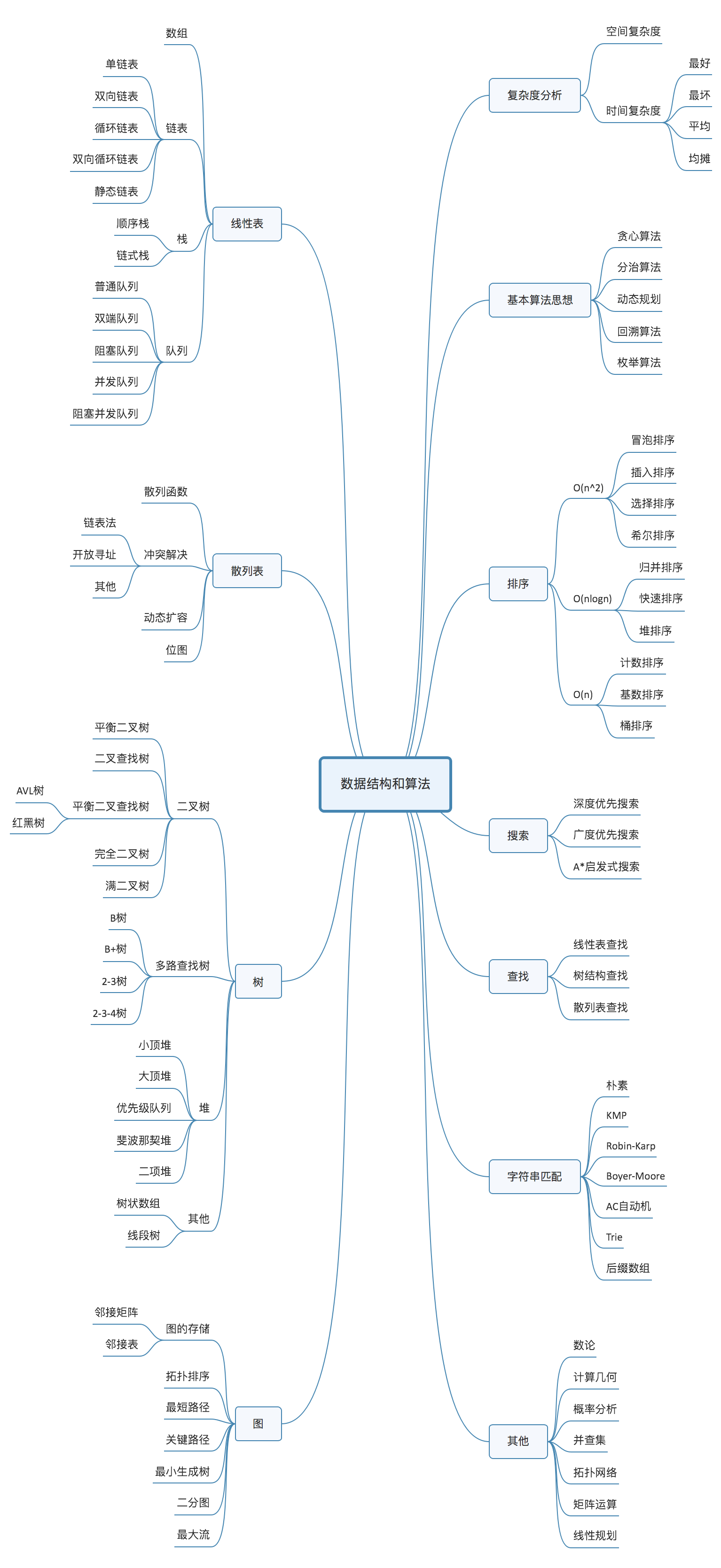# 2. 如何学习？

• 复杂度分析
• 数据结构与算法是解决如何更省，更快的存储和处理数据的问题
• 复杂度分析就是对应的考量效率和资源消耗的方法• 数据结构算法树状图
• 复杂度分析
• 空间复杂度
• 时间复杂度
• 最好
• 最坏
• 平均
• 均摊
• 基本算法思想
• 贪心
• 分治
• 动态规划
• 回溯
• 枚举
• 排序
• O(n^2)
• 冒泡排序
• 插入排序
• 选择排序
• 希尔排序
• O(nlogn)
• 归并排序
• 快速排序
• 堆排序
• O(n)
• 计数排序
• 基数排序
• 桶排序
• 搜索
• 深度优先搜索
• 广度优先搜索
• A启发式搜索
• 查找
• 线性表查找
• 树结构查找
• 散列表查找
• 字符串匹配
• 朴素
• KMP
• Robin-Karp
• Boyer-Moore
• AC自动机
• Trie
• 后缀数组
• 存储
• 邻接矩阵
• 邻接表
• 拓扑排序
• 最短路径
• 关键路径
• 最小生成树
• 二分图
• 最大流
• 二叉树
• 平衡二叉树
• 二叉查找树
• 平衡二叉查找树
• AVL树
• 红黑树
• 完全二叉树
• 满二叉树
• 多路查找树
• B 树
• B+ 树
• 2-3 树
• 2-3-4 树
• 小顶堆
• 大顶堆
• 优先级队列
• 斐波那契堆
• 二项堆
• 其他
• 梨形数组
• 线段树
• 散列表
• 散列函数
• 冲突解决
• 链表法
• 开放寻址
• 其他
• 动态扩容
• 位图
• 线性表
• 数组
• 链表
• 单链表
• 双向链表
• 循环链表
• 双向循环链表
• 静态链表
• 顺序栈
• 链式栈
• 队列
• 普通队列
• 双端队列
• 阻塞队列
• 并发队列
• 阻塞并发队列

## 2.1 最常用的基础总结

• 数据结构
• 数组
• 链表
• 队列
• 散列表
• 二叉树
• 跳表
• Trie树
• 算法
• 递归
• 排序
• 二分查找
• 搜索
• 哈希算法
• 贪心算法
• 分治算法
• 回溯算法
• 动态规划
• 字符串匹配算法

• 其自身来历
• 特点
• 适合解决的问题
• 实际的应用场景

×